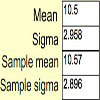#### You may also like### Spinners

How do scores on dice and factors of polynomials relate to each other?### Data Matching

Use your skill and judgement to match the sets of random data.### Random Inequalities

Can you build a distribution with the maximum theoretical spread?

# Time to Evolve 2

##### Age 16 to 18Challenge Level

What are the formulae for the expectation of a sum and the variance of a sum?

In creating the trial simulation, you could use the spreadsheet functions

RAND() returns a $\mbox{U}[0,1]$ random variable.

COUNTIF($A$5:$A$1005,"=96") counts the number of cells in the range$A$5:$A$1005 which equal the value 96